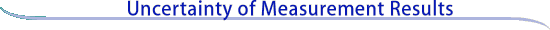Expanded uncertainty and coverage factor

Expanded uncertainty

Although the combined standard uncertainty uc is used to express the uncertainty of many measurement results, for some commercial, industrial, and regulatory applications (e.g., when health and safety are concerned), what is often required is a measure of uncertainty that defines an interval about the measurement result y within which the value of the measurand Y can be confidently asserted to lie. The measure of uncertainty intended to meet this requirement is termed expanded uncertainty, suggested symbol U, and is obtained by multiplying uc(y) by a coverage factor, suggested symbol k. Thus U = kuc(y) and it is confidently believed that Y is greater than or equal to y - U, and is less than or equal to y + U, which is commonly written as Y = y ± U.

Coverage factor

In general, the value of the coverage factor k is chosen on the basis of the desired level of confidence to be associated with the interval defined by U = kuc. Typically, k is in the range 2 to 3. When the normal distribution applies and uc is a reliable estimate of the standard deviation of y, U = 2 uc (i.e., k = 2) defines an interval having a level of confidence of approximately 95 %, and U = 3 uc (i.e., k = 3) defines an interval having a level of confidence greater than 99 %.

Relative expanded uncertainty

In analogy with relative standard uncertainty ur and relative combined standard uncertainty uc,r defined above in connection with simplified forms of equation (6), the relative expanded uncertainty of a measurement result y is Ur = U/|y|, y not equal to zero.

Continue to
Examples of uncertainty statements